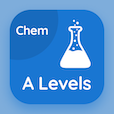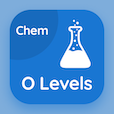Cambridge Online Courses (GCE)

A Level Chemistry MCQs

A Level Chemistry MCQ - Topics

Learn Number of Nucleons Multiple Choice Questions (MCQ Quiz), Number of Nucleons quiz answers PDF to learn a level chemistry course for a level chemistry online classes. Atomic Structure and Theory Multiple Choice Questions and Answers (MCQs), Number of Nucleons quiz questions for online schools that offer certificate programs. Number of Nucleons Book PDF: atom facts, number of nucleons, elements and atoms test prep for colleges that offer certificate programs.

"The atomic number is the number of" MCQ PDF: number of nucleons App APK with protons (p+), electrons (e-), neutrons (n0), and nucleons (p+ and n0) choices for online schools that offer certificate programs. Study number of nucleons quiz questions for merit scholarship test and certificate programs for free online college classes.

## MCQs on Number of Nucleons Quiz

MCQ: The atomic number is the number of

protons (p+)
electrons (e-)
neutrons (n0)
nucleons (p+ and n0)

MCQ: The sum of protons and neutrons in an atom gives off

nucleon number only
mass number only
atomic number
nucleon and mass number

MCQ: The elements which have the same number of protons but different number of neutrons are called

isotopes
isomers
allotropes
allomers

MCQ: The proton number is denoted by the symbol

A
Z
P
N

MCQ: Particular element posses the same number of electrons hence have the same

chemical properties
physical properties
density
volume

### More Quizzes on A Level Chemistry Book